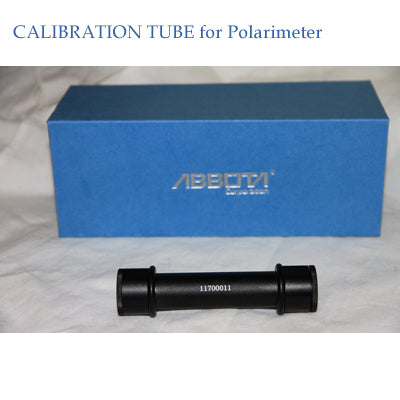SKU: PST-N17• Polarimeter Calibration tube
• Optical rotation: +17 or -17
• Sugar degree: -48.51'Z
• The reading is got using the sodium light (effective wave length is 589.44 nm) to perform the measurement at 20.0'C .
• Calculate temperature calibration according to the formula following: At 'C =A20.0'C [1+0.000144(t-20)] Where A20.0'C is the reading of standard quartz tube at 20.0'C ; At 'C is the reading of standard quartz tube at t'C